# Adding Fractions with Unlike Denominators

Times up!
Times up! You may finish answering the current question and then proceed to your score!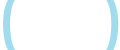Sorry, you have used all of your available hints for this lesson.
Practice Limit Reached

You've reached your daily practice limit of 12 questions.

Award InformationAwarded toQuestion HintChoose Family Member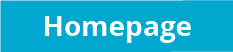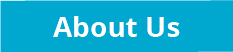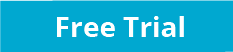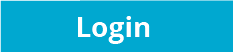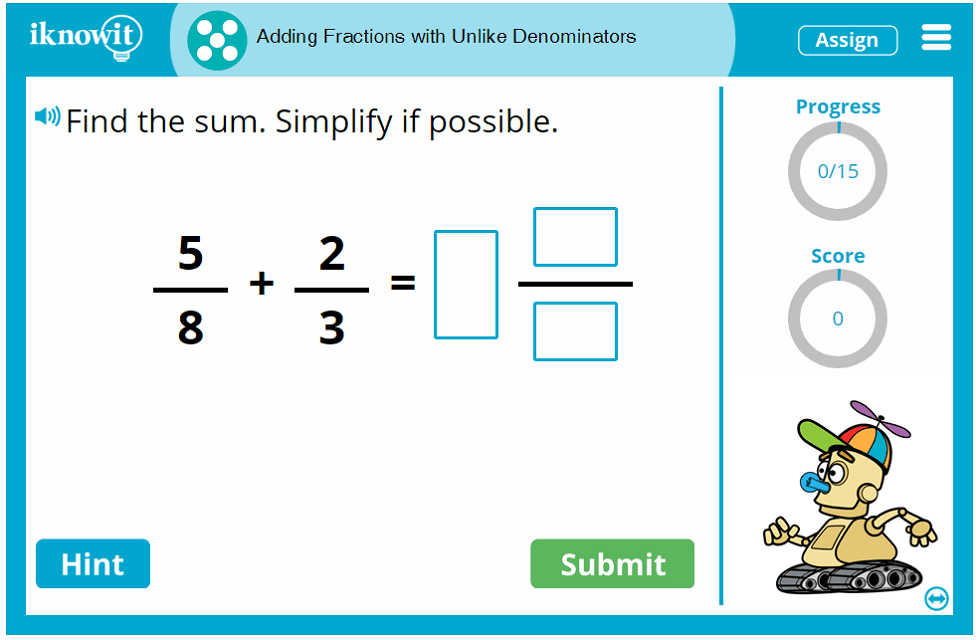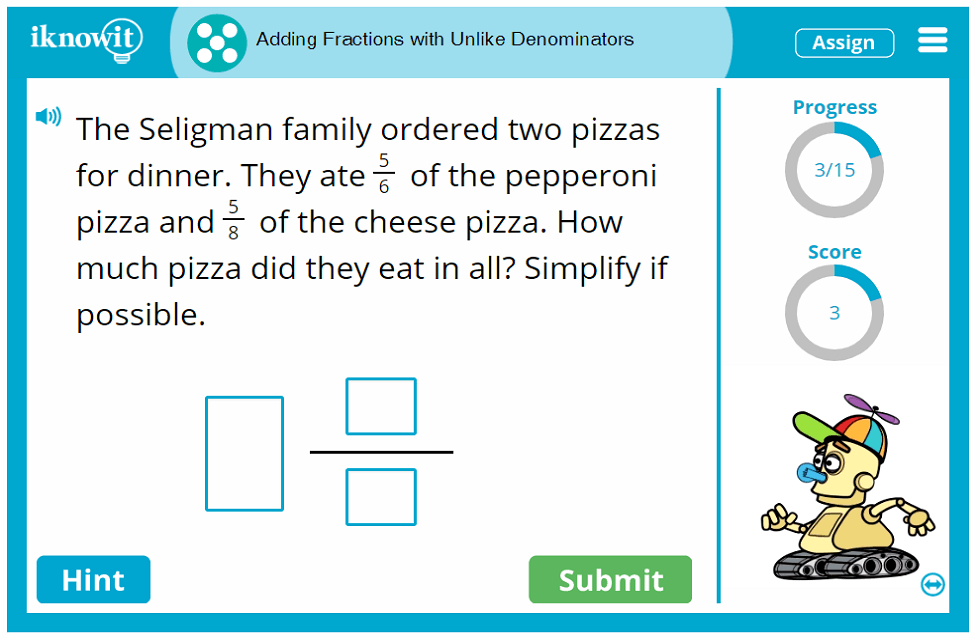## Interactive Math Activity - Adding Fractions with Unlike Denominators

Give your fifth-grade students an opportunity to practice adding fractions with unlike denominators in this engaging math activity from iKnowIt.com. Students will find the sum of two fractions with unlike denominators and simplify their answer if possible. Your students will practice finding a common denominator and writing equivalent fractions to solve each fraction addition problem. After completing this online math lesson, your class will be experts at adding fractions with unlike denominators.

Students will be engaged and challenged by the math problems in this online math game. Students may be asked to find the sum of 9/10 and 2/4. They may be required to find the sum of 2/9 and 6/7. Or they may need to solve a word problem like this one: "Trinity Elementary School is having a fundraiser at Pete's Pizza. 7/12 of Mr. Brandt's class and 7/9 of Mrs. Flaum's class came to the fundraiser. What fraction of their classes came to the fundraiser? Simplify if possible." If students get stuck on a question, they can click on the "Hint" button for a helpful clue. They will be prompted to find the common denominator and write equivalent fractions. When students answer a question incorrectly, they will be shown a detailed explanation page that reveals the correct answer and how to obtain it.

All math lessons on iKnowIt.com, including this fifth-grade fraction lesson, contain handy tools and features that help students make the most out of their math practice time. A progress-tracker in the upper-right corner of the practice screen shows students how many questions they have answered out of the total number of questions in the lesson. A score-tracker lets them see how many points they have earned so far. The speaker icon in the upper-left corner of the practice screen indicates the read-aloud feature; when students click the button, the question will be read out loud to them in a clear voice. This is a great resource for ESL/ELL students and students who are auditory processors.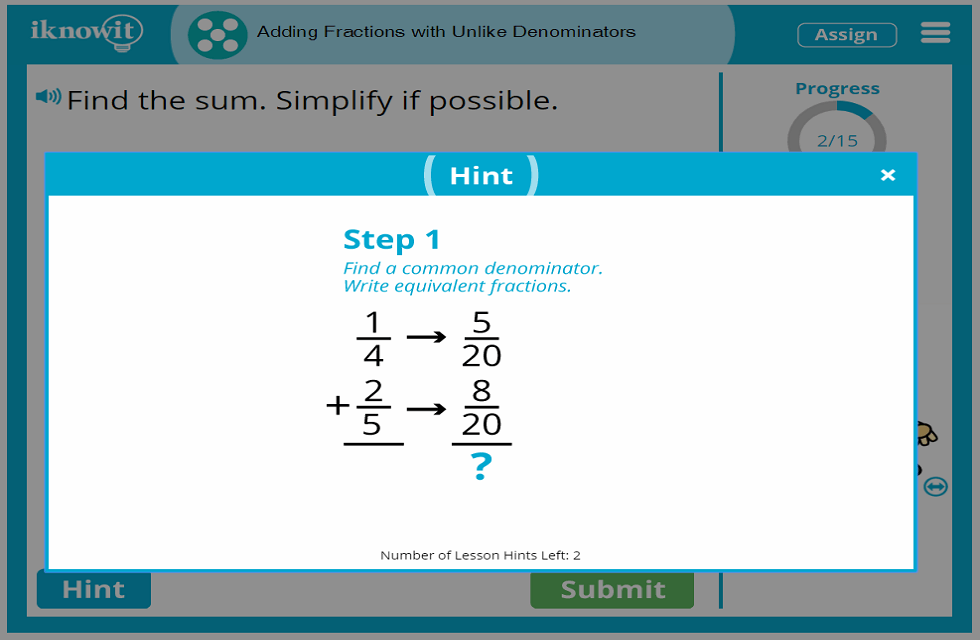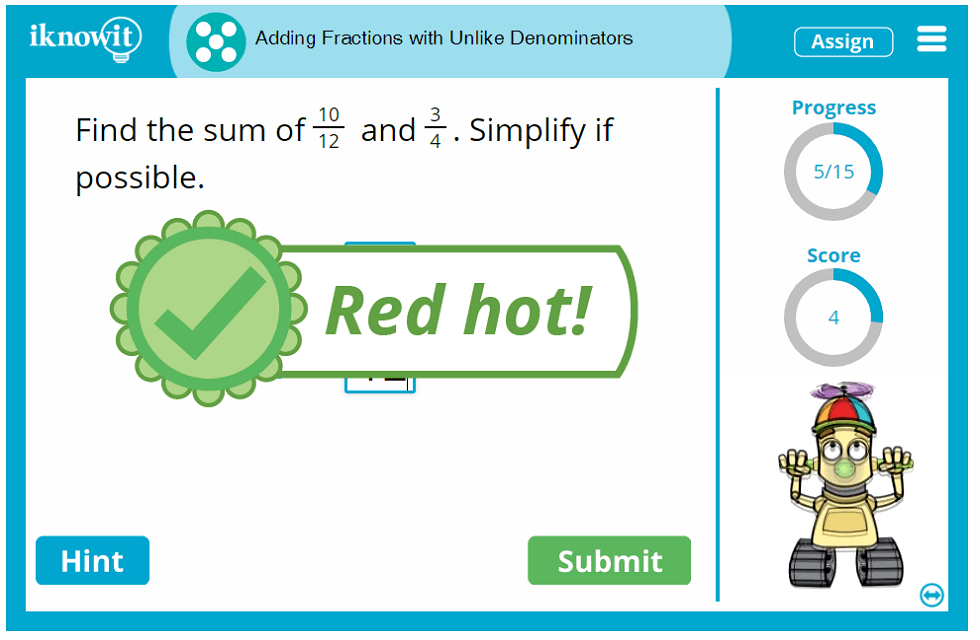## Why Choose 'I Know It'?

Teachers, homeschool educators, and students alike find our online math program helpful, engaging, and fun! Use the "I Know It" math practice program alongside your traditional math curriculum for extra practice and see for yourself the difference interactive math can make! Teachers and homeschool educators appreciate the wide variety of math lessons we offer, featuring the most popular math topics in kindergarten through fifth grade. All of our math lessons are aligned to the Common Core Standard and are arranged by grade level and topic, making it easy for you to find exactly what you need right when you need it. Students enjoy practicing math skills on iKnowIt.com because our lessons are engaging and fun. Whimsical animated characters cheer students on in their practice sessions, while positive feedback and the opportunity to earn math awards give students incentive to keep going. It's like a fun, yet challenging, math game!

We hope you will try out this fifth-grade fractions lesson with your students today! Remember to browse our extensive collection of math lessons for other topics your class will enjoy.

## Free Trial and Membership Information

id you know we offer a free sixty-day trial to anyone who is interested in trying out our math practice program? We do! In fact, when you sign up for a free trial, you can try out this fifth-grade math game, as well as every other math lesson on our website, at no cost! We’re confident you will love using "I Know It" with your students, so when your free trial runs out, we encourage you to go ahead and register as a member of "I Know It." This way, you can enjoy the benefits of interactive math practice for a full calendar year!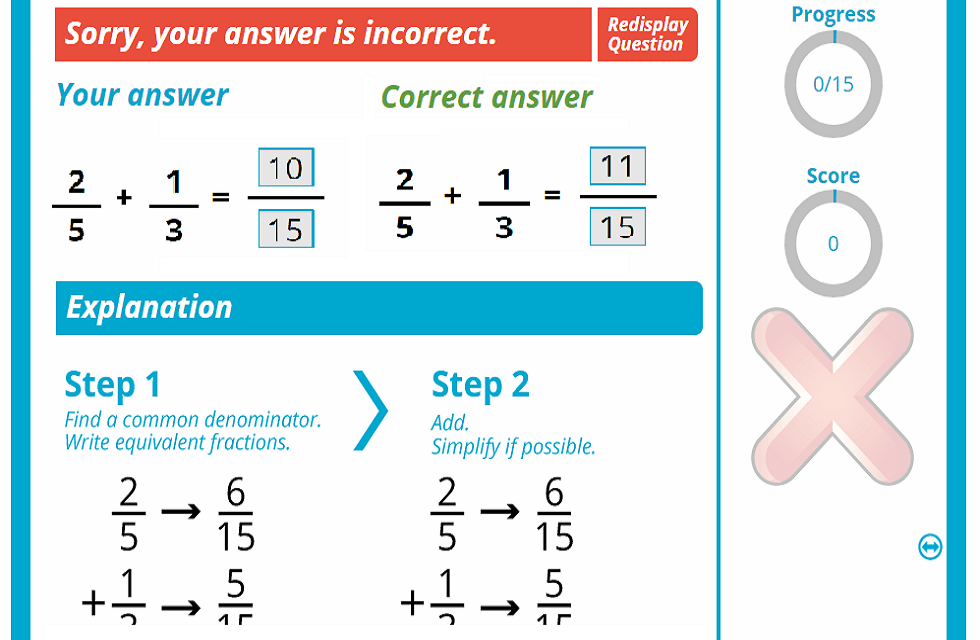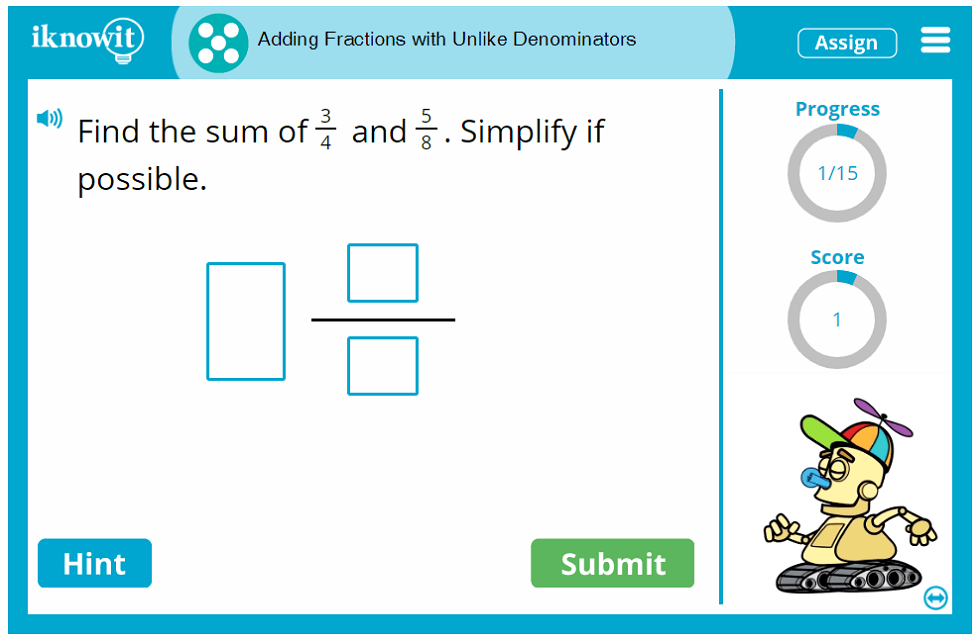## Level

This interactive math lesson is classified as Level E. It may be ideal for your fifth-grade class.

## Common Core Standard

5.NF.1
Numbers and Operations - Fractions

Students will use equivalent fractions as a strategy to add and subtract fractions. Students will demonstrate an ability to add and subtract fractions with unlike denominators by replacing given fractions with equivalent fractions.

## You might also be interested in...

Adding Mixed Numbers with Unlike Denominators (Level E)
In this fifth-grade level math lesson, students will practice adding mixed numbers with unlike denominators. Students will find the sum and simplify if possible.

Adding and Subtracting Fractions with Unlike Denominators (Level E)
Students will practice adding and subtracting fractions with unlike denominators in this interactive math lesson geared toward fifth grade. Students will find the sum or difference and simplify if possible.